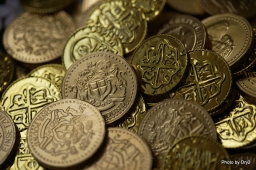# Five-crown 13621

Jana saves two-crown and five-crown coins. He has ten coins in the coffers. How many are two Czech crowns, and how many are five Czech crowns if he has saved CZK 29?

a =  7
b =  3

### Step-by-step explanation:

a+b=10
2a+5b=29

a+b=10
2·a+5·b=29

a+b = 10
2a+5b = 29

Pivot: Row 1 ↔ Row 2
2a+5b = 29
a+b = 10

Row 2 - 1/2 · Row 1 → Row 2
2a+5b = 29
-1.5b = -4.5

b = -4.5/-1.5 = 3
a = 29-5b/2 = 29-5 · 3/2 = 7

a = 7
b = 3

Our linear equations calculator calculates it.Did you find an error or inaccuracy? Feel free to write us. Thank you!

Tips for related online calculators
Do you have a system of equations and looking for calculator system of linear equations?